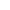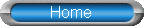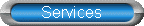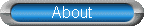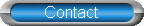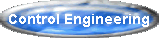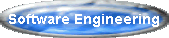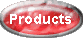Tarman Engineering, Inc. also markets products that it has developed.FPAIN is a simple, flexible, easy to use financial calculator program that performs calculations
utilizing the 5 core finance variables:  Future value, Present Value, Annuity value, Interest rate,
and Number of periods.

When 3 of these variables are set, FPAIN will automatically calculate the 2 remaining variables.
If both the Interest Rate and Number of Periods are set, then one of the Values must be set.
If 2 of the Values are set, either Interest Rate OR the Number of Periods must be set.